International
Tables for
Crystallography
Volume C
Mathematical, physical and chemical tables
Edited by E. Prince

International Tables for Crystallography (2006). Vol. C, ch. 1.3, pp. 10-12

Section 1.3.2. Twin lattices

E. Kocha

aInstitut für Mineralogie, Petrologie und Kristallographie, Universität Marburg, Hans-Meerwein-Strasse, D-35032 Marburg, Germany

1.3.2. Twin lattices

| top | pdf |

For reflection and rotation twins described in the last section, a special situation arises whenever there exists a lattice vector perpendicular to the twin plane or a lattice plane perpendicular to a rational twofold twin axis. Such a situation occurs systematically for all reflection and rotation twins with cubic symmetry and for certain twins with non-cubic symmetry (cf. Table 1.3.2.1). In addition, such a perpendicularity may occur occasionally if equation (1.1.2.12) is satisfied.

 Table 1.3.2.1| top | pdf | Lattice planes and rows that are perpendicular to each other independently of the metrical parameters
Basis systemLattice plane (hkl)Lattice row [uvw]Perpendicularity condition
Triclinic
Monoclinic (unique axis b) (010) 
Monoclinic (unique axis c) (001) 
Orthorhombic (100) 
(010) 
(001) 
Hexagonal/trigonal (hk0) [uv0] u = 2h + k, v = h + 2k
(001) 
Rhombohedral (h, k, −hk) [u, v, −uv] u = h, v = k
(111) 
Tetragonal (hk0) [uv0] u = h, v = k
(001) 
Cubic (hkl) [uvw] u = h, v = k, w = l

In the case of a noncentrosymmetric crystal structure, different twins result from a twin axis [uvw] with a perpendicular lattice plane (hkl), or from a twin plane (hkl) with a perpendicular lattice row [uvw]: the reflection twin consists of two enantiomorphous twin components whereas the rotation twin is built up from two crystals with the same handedness (cf., for example, Brazil twins and Dauphiné twins of quartz). With respect to the first twin component, the lattice of the second component has the same orientation in both cases. For a centrosymmetrical crystal structure, both twin laws give rise to the same twin.

Whenever a twin plane or twin axis is perpendicular to a lattice vector or a net plane, respectively, the vector lattices of the twin components have a three-dimensional subset in common. This sublattice [derivative lattice, cf. IT A (2005, Chapter 13.2)] is called the twin lattice. It corresponds uniquely to the intersection group of the two translation groups referring to the twin components. The respective subgroup index i is called the twin index. It is equal to the ratio of the volumes of the primitive unit cells for the twin lattice and the crystal structure. If one subdivides the crystal lattice into nets parallel to the twin plane or perpendicular to the twin axis, each ith of these nets belongs to the common twin lattice of the two twin components (cf. Fig. 1.3.2.1). Important examples are cubic twins with  as twofold twin axis or (111) as twin plane and rhombohedral twins with  as twin axes or (001) as twin plane (hexagonal description). In all these cases, the twin index i equals 3.Figure 1.3.2.1 | top | pdf |(a) Projection of the lattices of the twin components of a monoclinic twinned crystal (unique axis c, γ = 93°) with twin index 3. The twin may be interpreted either as a rotation twin with twin axis  or as a reflection twin with twin plane (110). (b) Projection of the corresponding reciprocal lattices.

For every twin lattice, its twin index i can be calculated from the Miller indices of the net plane (hkl) and the coprime coefficients u, v, w of the lattice vector t perpendicular to (hkl). Referred to a primitive lattice basis, i is simply related to the modulus of the scalar product j of the two vectors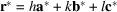and: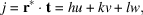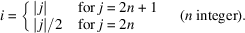The same procedure – but with modified coefficients – may be applied to a centred lattice described with respect to a conventionally chosen basis: The coprime Miller indices h, k, l that characterize the net plane have to be replaced by larger non-coprime indices h′, k′, l′, if h, k, l do not refer to a (non-extinct) point of the reciprocal lattice. The integer coefficients u, v, w specifying the lattice vector perpendicular to (hkl) have to be replaced by smaller non-integer coefficients u′, v′, w′, if the centred lattice contains such a vector in the direction [uvw].

1.3.2.1. Examples

| top | pdf |

• (1) Cubic P lattice:  is perpendicular to (111).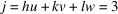odd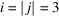.

• (2) Orthorhombic lattice with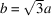:  is perpendicular to (110).

• (i) P lattice (cf. Fig. 1.3.2.2):Figure 1.3.2.2 | top | pdf |Projection of the lattices of the twin components of an orthorhombic twinned crystal (oP, b =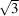a) with twin index 2. The twin may be interpreted either as a rotation twin with twin axis  or as a reflection twin with twin plane (110). The figure shows, in addition, that twin index 1 results if the oP lattice is replaced by an oC lattice in this example (twinning by pseudomerohedry).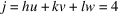even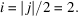• (ii) C lattice (cf. also Fig. 1.3.2.2):

Because of the C centring,  has to be replaced by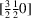.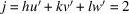even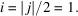• (3) Orthorhombic C lattice with b = 2a:  is perpendicular to (120) (cf. Fig. 1.3.2.3).Figure 1.3.2.3 | top | pdf |Projection of the lattices of the twin components of an orthorhombic twinned crystal (oC, b = 2a) with twin index 4. The twin may be interpreted either as a rotation twin with twin axis  or as a reflection twin with twin plane (120).

As (120) refers to an extinct reflection' of a C lattice, the triplet 240 has to be used in the calculation.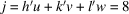even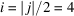.

• (4) Rhombohedral lattice in hexagonal description with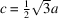: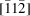is perpendicular to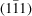.

Because of the R centring,has to be replaced by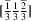.

Asrefers to an extinct reflection' of an R lattice, the triplet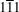has to be replaced by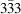.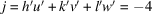evenReferences

International Tables for Crystallography (2005). Vol. A, Space-group symmetry, edited by Th. Hahn. Heidelberg: Springer.